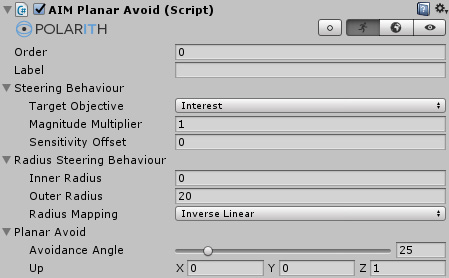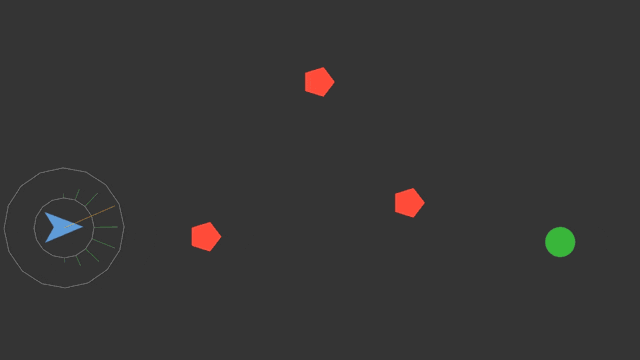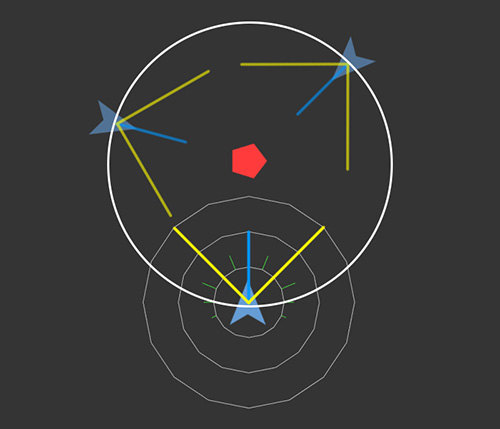1.7
Planar Avoid

front-end / back-end: `AIMPlanarAvoid` | `PlanarAvoid`
inherits from: `AIMRadiusSteeringBehaviour` | `RadiusSteeringBehaviour`In certain situations, it might be not enough to sample potential dangers in the world, so your agent has to avoid such objects actively. Instead of writing a specific amount of danger objective values, this behaviour calculates a possible avoidance direction. Thus, it is intended to write these values into an objective representing interest. The premise of this behaviour is that the agent moves in a plane defined by `Up`. Hence, it can avoid left or right and so, this behaviour is a planar behaviour which requires proper projection settings for 3D scenarios.

## Properties

This component has got the following specific properties.

Property Description
`AvoidanceAngle` This angle defines the possible avoid direction (for both left and right) with respect to an agent's movement direction.
`Up` Needed to calculate the avoid directions. `Up` is the normal of the plane the agent moves in.

## Preview## Remarks

This behaviour requires valid velocities. So, if an agent has no Rigidbody attached, a Steering Tag which has `TrackVelocity` activated needs to be present.

The effect of Planar Avoid is shown in the following figure.Figure 1: Shows how the Planar Avoid behaviour influences objective values. The greatest magnitudes occur along directions resulting from the given `AvoidanceAngle` (yellow). However, magnitudes decrease if the direction faces the obstacle. Depending on the agent's position relative to the obstacle, the optimal avoidance direction would be the tangent of the illustrated circle.

### Avoidance Angle

Let's have a closer look on how the `AvoidanceAngle` works. For example, if the direction would be (0, 0, 1), the `Up` vector would be (0, 1, 0) and the avoid angle would be 45°, the resulting directions would be approximately (-0.7, 0, 0.7) and (0.7, 0, 0.7). Note, this parameter is highly dependent on your controller. For a direct controller, a smaller value (~0 to ~5) might be a better choice, as against a physics-based controller normally benefits from a larger angle (~20 to ~40) in order for the applied forces to have an impact on the Rigidbody(2D).

### Projection Mode

In theory, it is possible to apply this behaviour for arbitrary agent orientations. However, this behaviour works in a planar manner which means that the obstacle should always be located in the agent's perceptional plane (defined by the `Up` vector). This can be ensured by using the correct projection mode: `PlaneXY` corresponds to `Up` = (0, 0, 1) and `PlaneXZ` corresponds to `Up` = (0, 1, 0).

### Mapping Type

Depending on your setup, either `InverseQuadratic` or `InverseSquareRoot` mappings might be useful instead of just using the simple `InverseLinear` mapping. In this way, for example, you can make your agents react early to suddenly occurring threats.